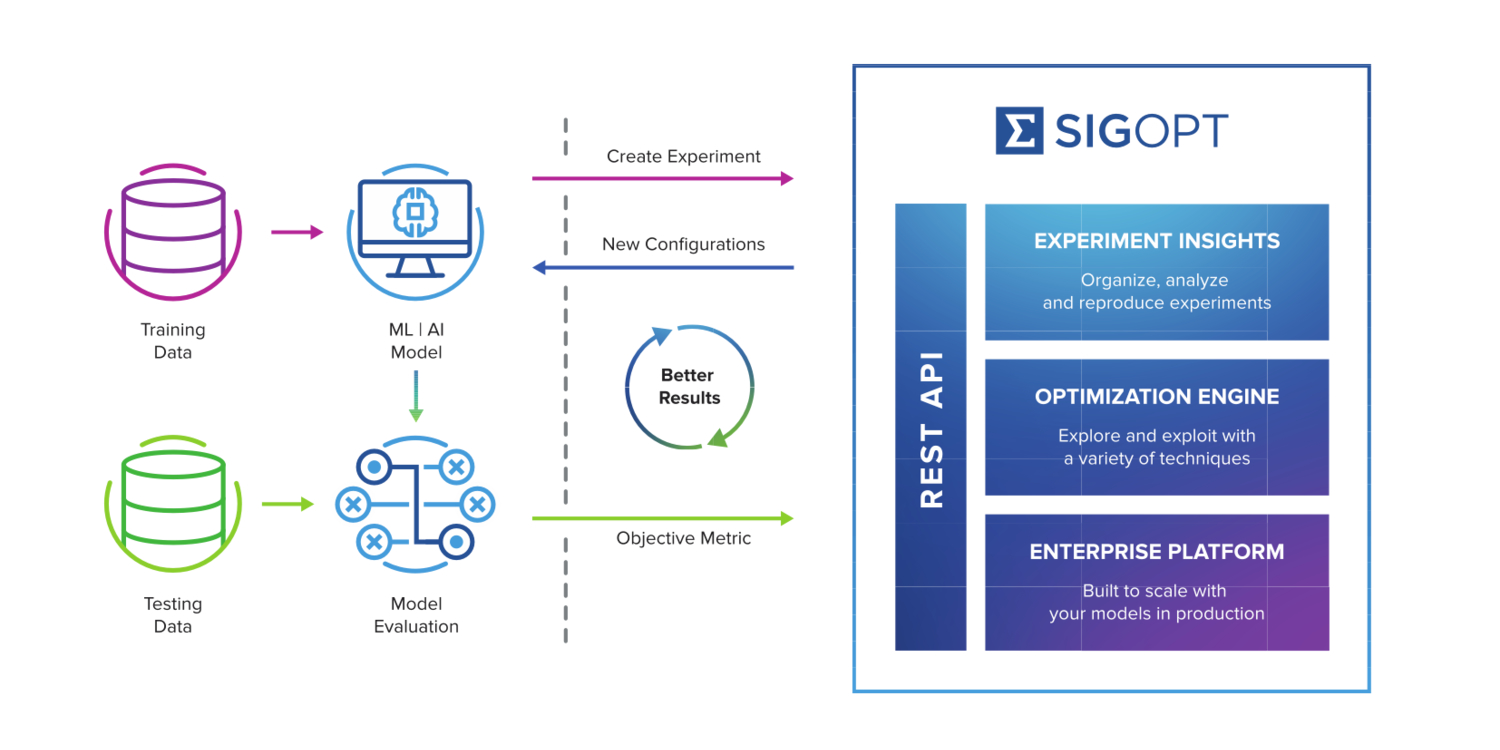# Automating model tuning and hyperparameter optimization with SigOpt

By guest author Nick Payton, Head of Marketing and Partnerships, SigOpt

The quality of predictions from machine learning (ML) models depends on a few factors. These factors include a high volume of well-prepared data, a robust feature set with the appropriate architecture, and the configuration of hyperparameters.

Hyperparameters are numeric values with high and low boundaries that dictate how a model assesses data to make a prediction. Hyperparameter optimization describes the process of efficiently searching for and identifying the most accurate configuration of hyperparameters for any given model. Whereas most model parameters are discovered by training models with data, hyperparameters – as well as some configuration parameters – cannot be discovered through this training process. Some common hyperparameters are learning rate, neurons, hidden layers, and choice of activation function. This Amazon SageMaker documentation explains more about how hyperparameter tuning works.

In this post, I show how to automate hyperparameter tuning, also known as optimization. This can accelerate the model development process and produce better outcomes.

## Overview

The ML process involves the following four stages:

1. Preparing data with data transformation, cleaning, and annotation
2. Engineering and analyzing features through a data science process
3. Analyzing and selecting the right model or model architecture
4. Training and tuning the model to improve performance

The challenge is that the cost of tuning your models increases with the complexity, volume, and variety of models in development. The model is trained based on the value that an expert specifies for each hyperparameter. Optimizing the configuration of these values becomes exponentially more challenging as the number of hyperparameters increases.

If configured appropriately, these hyperparameters improve the quality of a model’s output, which is typically measured by calculating prediction accuracy on unseen data. Hyperparameter optimization is essential to deploying effective ML models. However, teams often neglect it in the modeling process or relegate it to the end or the “last mile” of the development process. Doing so significantly limits its impact on model development.

I detail the challenges of popular model-tuning approaches in Hyperparameter Optimization on AWS with SigOpt. I demonstrate SigOpt’s optimization solution in the blog post, Fast CNN Tuning with AWS GPU Instances and SigOpt.

## SigOpt’s optimization approach

SigOpt’s model experimentation and optimization solutions provide a variety of learning methods, including Bayesian and global optimization algorithms. This automated approach to experimentation provides you with insights on your models and experiments through your dashboard. By replacing exploration of potential models with better-performing model configurations, you can find optimal configurations by learning from previous attempts.

This approach transforms ML model development into a prototyping process. You can use SigOpt’s software as a service (SaaS) solution to automate tuning, accelerate development, and amplify model performance. This approach to optimization does not require access to customer data or models for tuning, so you maintain security and privacy.

With each model configuration tracked in your dashboard, you do not have to worry about reproducibility. If you use multiple clouds or both cloud and on-premises infrastructure, you can use SigOpt across all your environments for a consistent user experience.

The following diagram presents a simplified version of how SigOpt’s API-enabled solution works.## Tuning hyperparameters for ML models using SigOpt

Following are the four steps to implement SigOpt and tune hyperparameters for any ML model. You’ll use this same process for any framework, including PyTorch, TensorFlow, or MXNet, among others.

These procedures are an example of how an ML engineer could use SigOpt directly in a Python notebook. You can customize your use of the SigOpt API to your needs. The API can be used with any model, library, or notebook.

For my example, I use this API in the context of optimizing a deep learning model using Keras and training the model with MNIST.

Before you begin, navigate to the SigOpt product page directly or search for SigOpt in AWS Marketplace (ML category) and subscribe. Then do the following:

1.      Install the SigOpt Client Library using the following code:

``````pip install sigopt

# Pass your API Token directly, overriding any environment variables
from sigopt import Connection
conn = Connection(client_token="YOUR_CLIENT_TOKEN_HERE")
``````

2.      Create the experiment using the following code:

``````experiment = conn.experiments().create(
name="Multi-Layer Perceptron",
parameters=[
dict(name="log_learning_rate", bounds=dict(min=-7, max=0), type="double"),
dict(
name="activation",
categorical_values=[
dict(name="relu"),
dict(name="sigmoid"),
dict(name="tanh"),
],
type="categorical",
),
dict(name="num_hidden_1", bounds=dict(min=1, max=6), type="int"),
dict(name="num_hidden_2", bounds=dict(min=1, max=6), type="int"),
dict(name="num_hidden_3", bounds=dict(min=1, max=6), type="int"),
],
template="python_keras_mlp"
),
observation_budget=70,
)
``````

3.      Parameterize the model using the following code:

``````import keras
from keras.models import Sequential
from keras.layers import Dense
from keras import optimizers

def create_model(assignments, x_train, y_train):
model = Sequential()
activation=assignments['activation']))
activation=assignments['activation']))
activation=assignments['activation']))
model.compile(
optimizer=optimizers.RMSprop(lr=10 ** assignments['log_learning_rate']),
loss='categorical_crossentropy',
metrics=['accuracy'],
)

model.fit(x_train, y_train, epochs=20, batch_size=32)
return model

from keras.datasets import mnist

(x_train, y_train), (x_test, y_test) = mnist.load_data()

x_train = x_train.reshape(x_train.shape, 784)
x_test = x_test.reshape(x_test.shape, 784)

y_train = keras.utils.to_categorical(y_train, num_classes=10)
y_test = keras.utils.to_categorical(y_test, num_classes=10)

def evaluate_model(assignments):
model = create_model(assignments, x_train, y_train)
return model.evaluate(x_test, y_test)
``````

4.      Run the optimization loop using the following code:

``````for _ in range(experiment.observation_budget):
suggestion = conn.experiments(experiment.id).suggestions().create()
assignments = suggestion.assignments
value = evaluate_model(assignments)
conn.experiments(experiment.id).observations().create(
suggestion=suggestion.id,
value=value,
)

best_assign = conn.experiments(experiment.id).best_assignments().fetch().data.assignments
# This is a SigOpt-tuned model
classifier = create_model(best_assign)
``````

SigOpt also has a gallery that includes a few additional packages that are available on its GitHub repository and within its API docs, including:

## Conclusion and next steps

In this post, I showed you how to automate hyperparameter tuning using SigOpt in order to help accelerate your development process. To learn more about the product or, visit the following resources:

This solution is available in AWS Marketplace. SigOpt is an AWS Machine Learning Competency Partner.Nick Payton is the Head of Marketing and Partnerships for SigOpt and loves spending his free time in the great outdoors.

The content and opinions in this post are those of the third-party author, and AWS is not responsible for the content or accuracy of this post.